Instant Maths Help:

# Edexcel A Level

## Modulus Function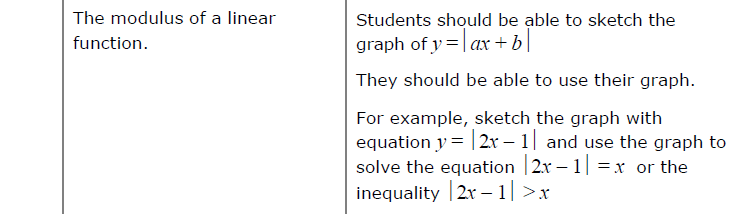##### Edexcel 9MA0/02 Jun 2023 A2 Exam Q. 12 :   10 marks in 12:00 min.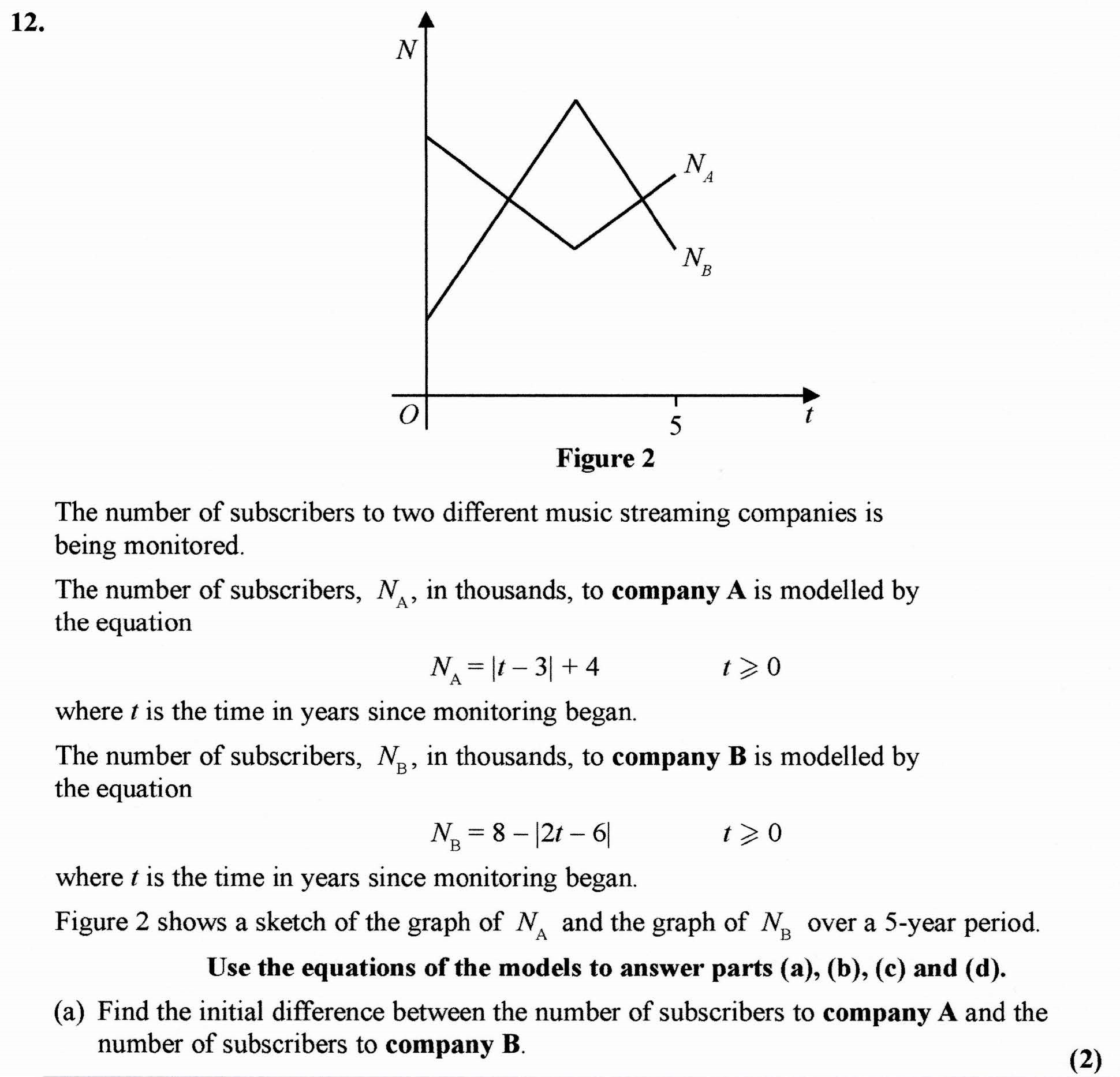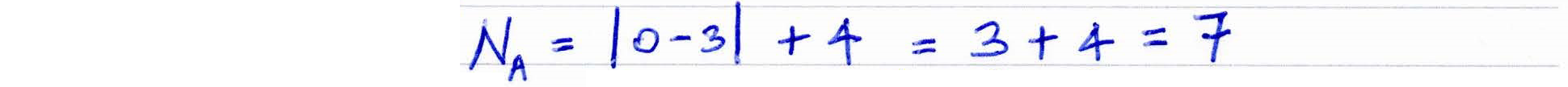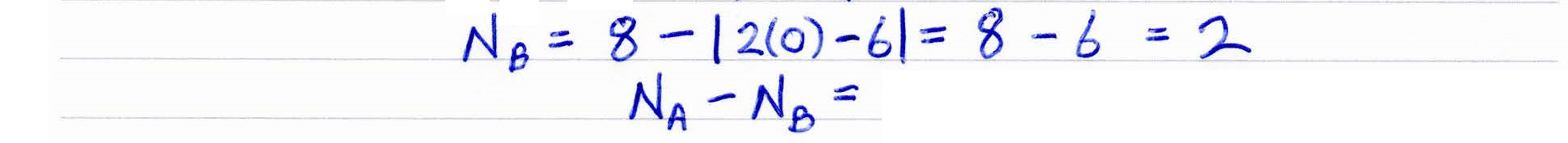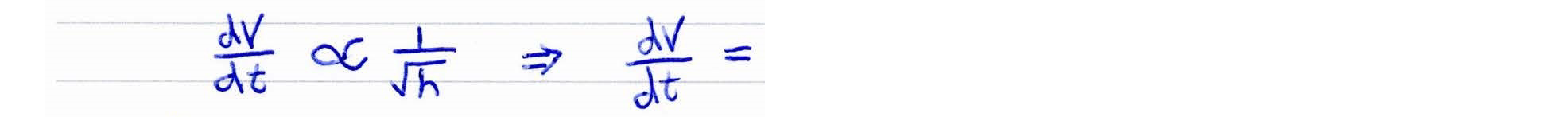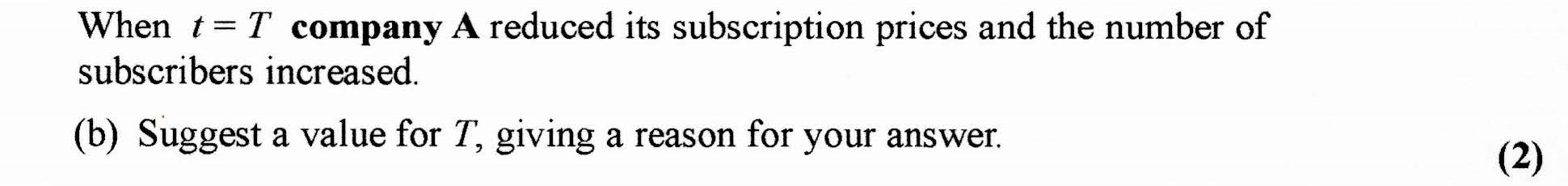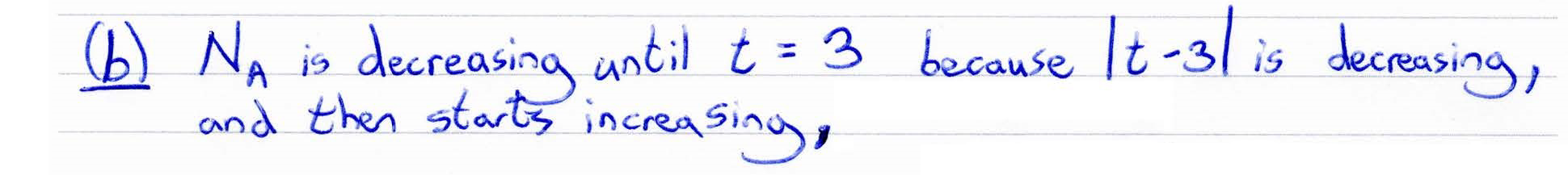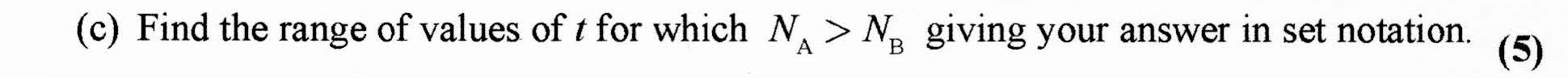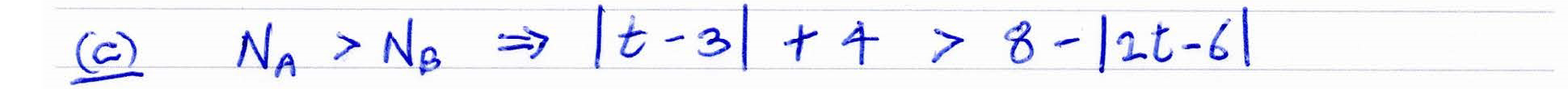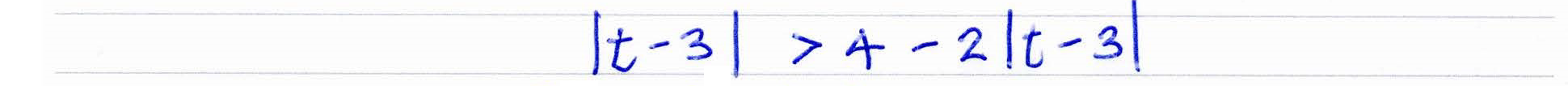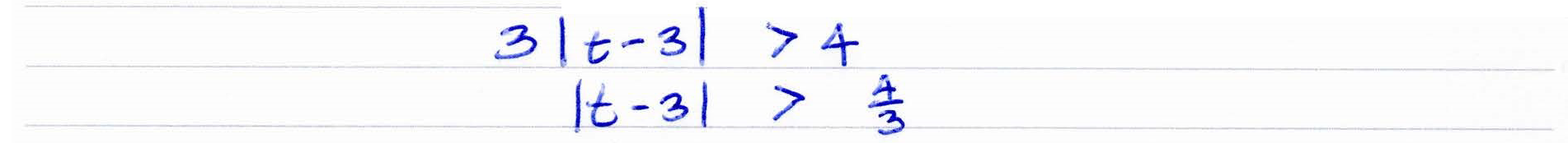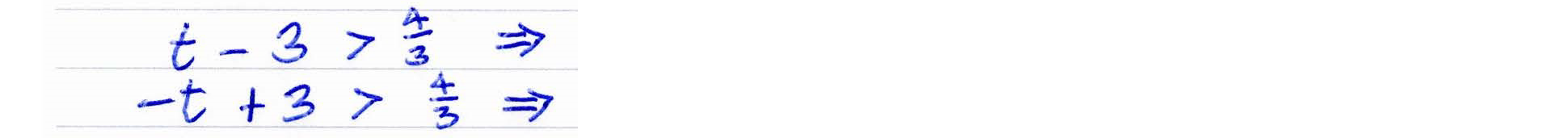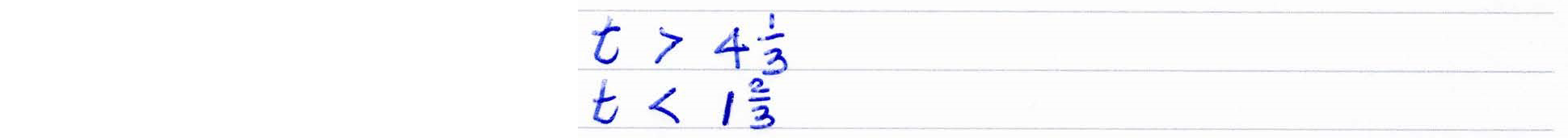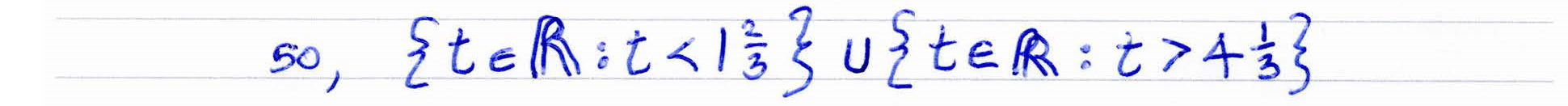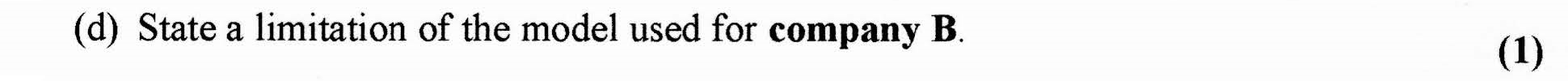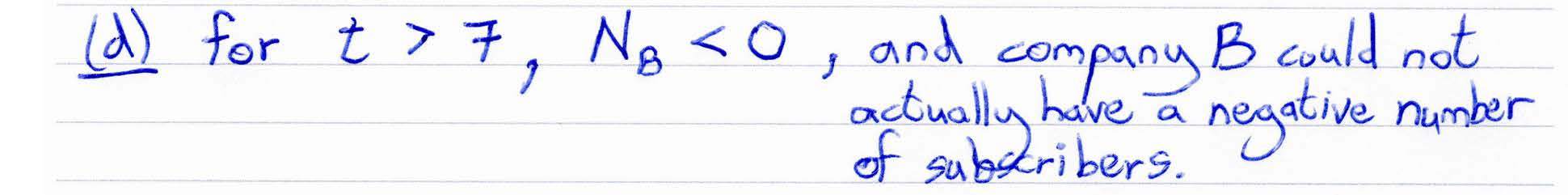##### Using modulus functions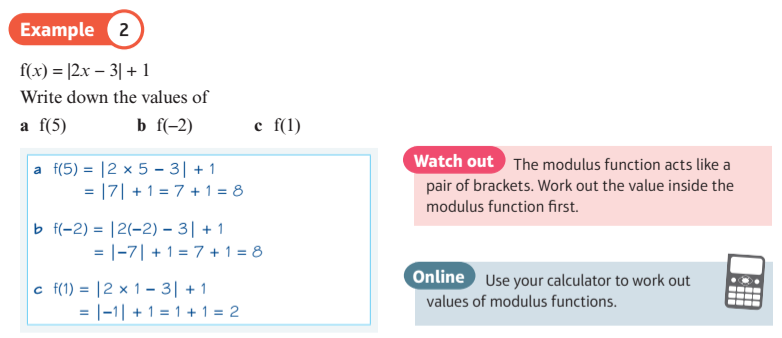##### Explore the solution to an inequality of a modulus function.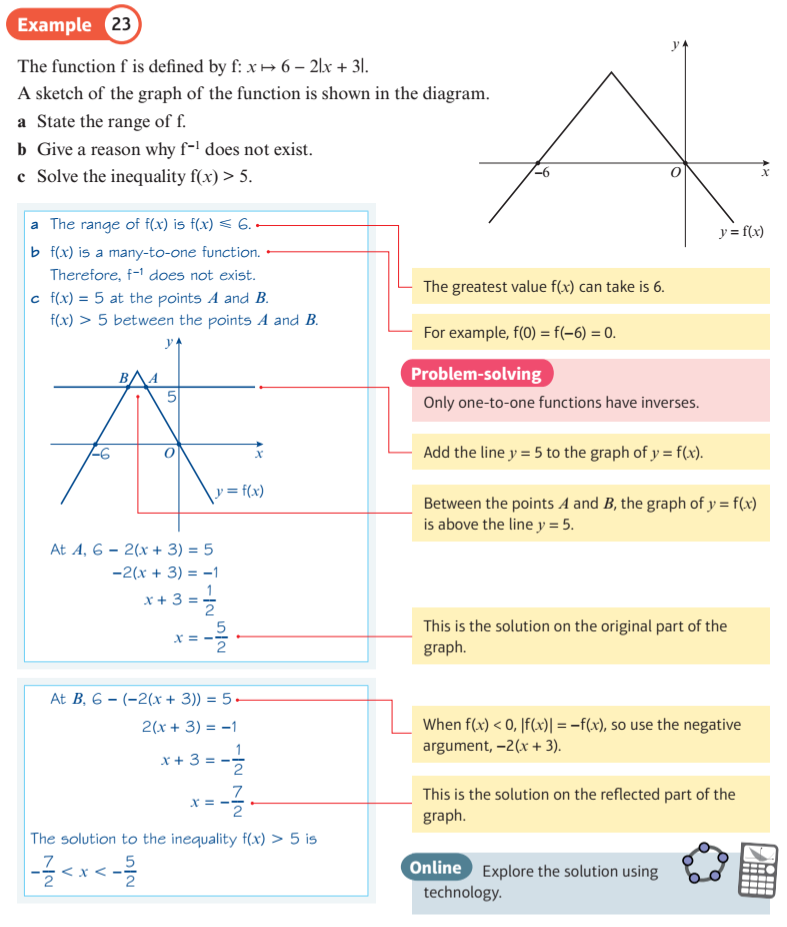##### Modulus of Line: y = | mx + c |
The graph of $$y = \left| {mx + c} \right|$$
##### Modulus of Line: y = | m (x + c) |
The graph of $$y = \left| {m(x + c)} \right|$$
##### Investigate Modulus Inequalities 1
The modulus inequality ${ a \, { x ^ 2 } + b \, x + c > \left| \, d \, x + e \, \right| }$ can be investigated in this display.

$\color{blue}{ f(x) = a \, { x ^ 2 } + b \, x + c }$ is shown in blue and $\color{red}{g(x) = \left| \, d \, x + e \, \right| }$ is shown in red.
The solution is values of $x$ for which $\color{blue}{f(x)}$ lies above $\color{red}{g(x)}$.
##### Investigate Modulus Inequalities 2
The solutions to quadratic modulus inequalities like $\color{green}{\left| \, a \, {x^2} + b \, x + c \, \right| > d \, x + e}$ can be illustrated graphically.

Both the curve $\color{blue}{y = \left| \, a \, {x^2} + b \, x + c \, \right|}$ and the line $\color{red}{y = d \, x + e}$ are plotted, and the inequality holds where the modulus of the curve lies above the line.

The solution is shown on the number line at the bottom. Note that the solution is itself an inequality, but in terms of just x. Note also, that intersection points are shown as open circles on the number line, because they are not included in the solution.
##### Modulus of Line: y = m |x| + c
The graph of $$y = m\left| x \right| + c$$